Function Repository Resource:

# SmootherStep

An improved sigmoidal interpolation function

Contributed by: Flip Phillips (FlipPhillips.com)
 ResourceFunction["SmootherStep"][x] returns a sigmoidal, Hermite interpolation between [0,1] for x on [0,1]. ResourceFunction["SmootherStep"][x,{min,max}] returns a sigmoidal, Hermite interpolation between [0,1] for x on [min,max].

## Details and Options

ResourceFunction["SmootherStep"] is an interpolation function commonly used in computer graphics.
ResourceFunction["SmootherStep"][x] clamps to 0 or 1 for x less than or greater than 0 or 1, respectively.
The original Perlin smoothstep is not second-order continuous at either x=0 or x=1. This creates discontinuities. ResourceFunction["SmootherStep"] solves this problem.

## Examples

### Basic Examples (2)

The function is sigmoidal, and inputs less than 0.5 underestimate the input:

 In:=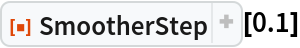Out=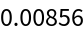Values greater than 0.5 do the opposite:

 In:=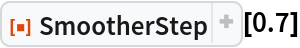Out=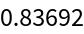Plot the function:

 In:=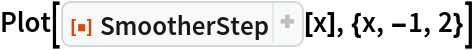Out=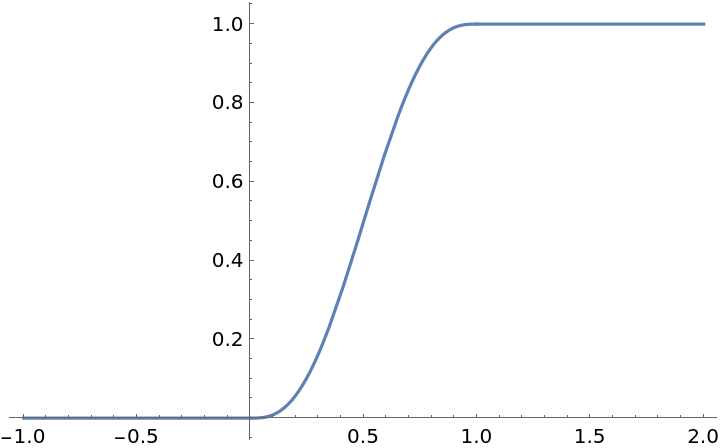### Scope (2)

The single-argument form of SmootherStep passes through the point {0.5,0.5}:

 In:=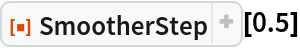Out=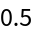Specify an input domain by giving a second argument:

 In:=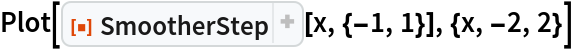Out=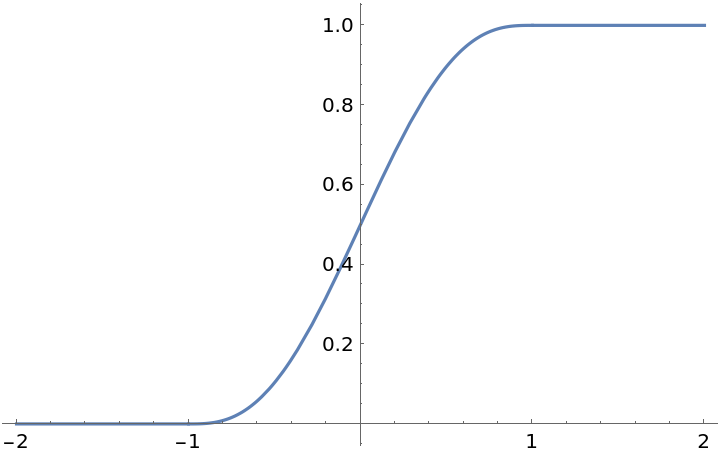### Applications (2)

Smoothly interpolate with more gradual changes at the start and stop of the interpolation domain:

 In:=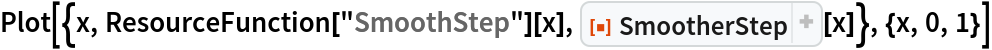Out=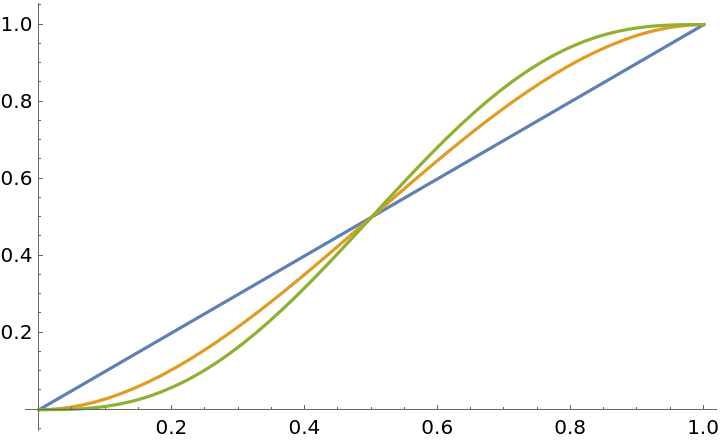Interpolate colors:

 In:=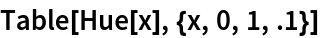Out=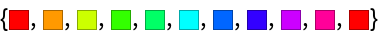In:=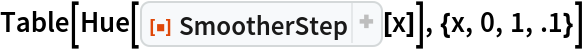Out=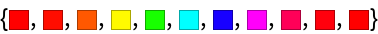### Properties and Relations (1)

The resource function SmoothSkip is second-order discontinuous at x=0 and x=1, creating artifacts in some uses. SmootherStep addresses this:

 In:=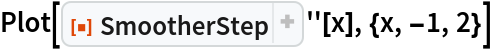Out=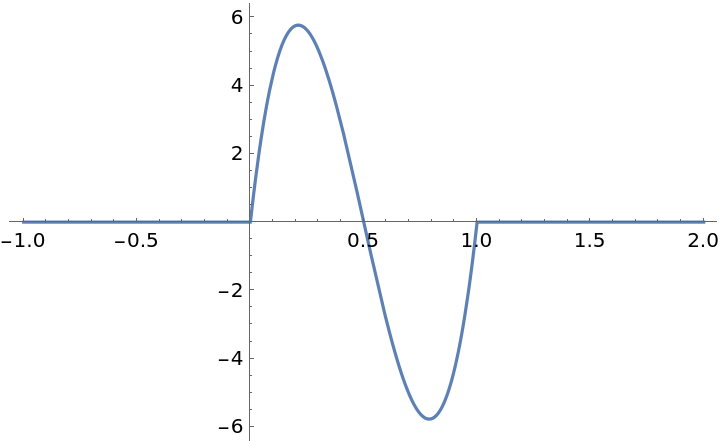### Neat Examples (1)

SmootherStep approximates the animation technique of "ease-in / ease-out":

 In:=Out=Flip Phillips

## Version History

• 1.0.0 – 08 August 2019